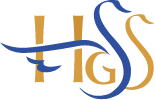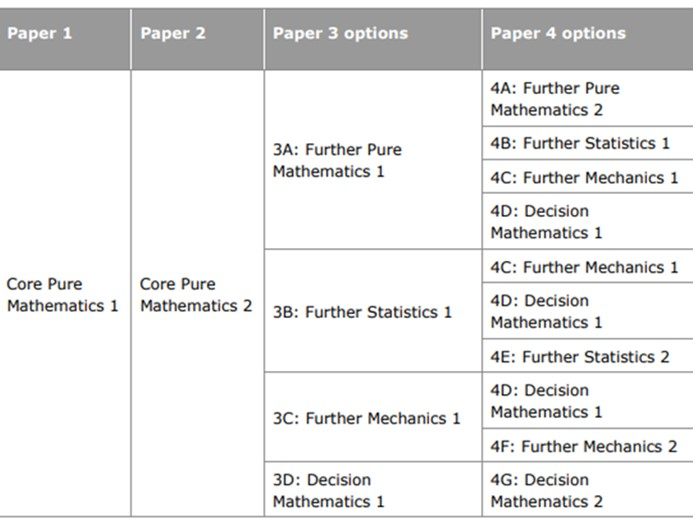# Further Mathematics - A Level

This course is suitable for those students who would like to significantly deepen and broaden their knowledge of Mathematics. Students will need to be passionate about the subject and have the ability to work independently.  Further Maths qualification enables students to understand mathematics and mathematical processes in ways that promote confidence, foster enjoyment and provide a strong foundation for progress to further study.

The course connects all the different areas of mathematics to other fields of study and allows the student to be aware of the relevance of mathematics to the world of work. A qualification in Further Maths is highly valued as indicator of intellectual ability, and unquestionably for mathematically related degrees at top universities.

## Course Content

### Year 12

Pure Mathematics 1 and 2

Algebraic techniques, 2-D Geometry, Graph Sketching, Sequences and an Introduction to Calculus. Vectors, Statistics and Mechanics. Trigonometry, Logs and Exponentials, Binomial Expansion and Geometrics Sequences and Series. Functions, Numerical Methods, Differentiation, Proofs and Further Trigonometry. Exponential and logarithms, Vectors. Advanced Trigonometry, Algebra and Calculus, Numerical Methods, Sequence and Series.

Statistics and Mechanics 1 and 2

Statistical sampling, Data presentation and interpretation, Probability, Kinematics, Forces and Newton’s Law. Further probability of significance testing. Kinematics, Forces, Moments.

### Year 13

Core Mathematics 1 and 2

Proof, Complex numbers, Matrices, Further Algebra and Functions, Further Calculus, Further Vectors, Polar

Coordinates, Hyperbolic Functions, Differential Equations

Options are subject to staff availability.

Students will be directed towards their strengths:•  Further Pure Mathematics 1 and 2
•  Further Statistics 1 and 2
•  Further Mechanics 1 and 2
•  Decision Mathematics 1 and 2

## Examinations

### Exam Board: Pearson Edexcel

2 Core Pure Mathematics Papers (25% each paper)

2 Options Papers (25% each paper)Head of subject: Mr J Ortega (KS5 Maths  Coordinator)